# How to Convert Square Yards to Square Inches | Area Units

The image below represents square yards to square inches.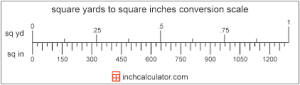To convert square yards to square inches, one essential parameter is needed and this parameter is Unit Value.

The formula for converting square yards to square inches:

1 Square Yard = 1296 Square Inches

Let’s solve an example;
Find the conversion of square yards to square inches when the unit value is 3.

1 Square Yard = 1296 Square Inches

Then,

3 Square Yards = (3 x 1296) Square Inches
3 Square Yards = 3888 Square Inches

Therefore, the square inch(es) is 3888.

Nickzom Calculator – The Calculator Encyclopedia is capable of converting square yards to square inches.

To get the answer and workings of square yards to square inches using the Nickzom Calculator – The Calculator Encyclopedia. First, you need to obtain the app.

You can get this app via any of these means:

You can also try the demo version via https://www.nickzom.org/calculator

Apple (Paid) – https://itunes.apple.com/us/app/nickzom-calculator/id1331162702?mt=8
Once, you have obtained the calculator encyclopedia app, proceed to the Calculator Map, then click on Area Units under Switches.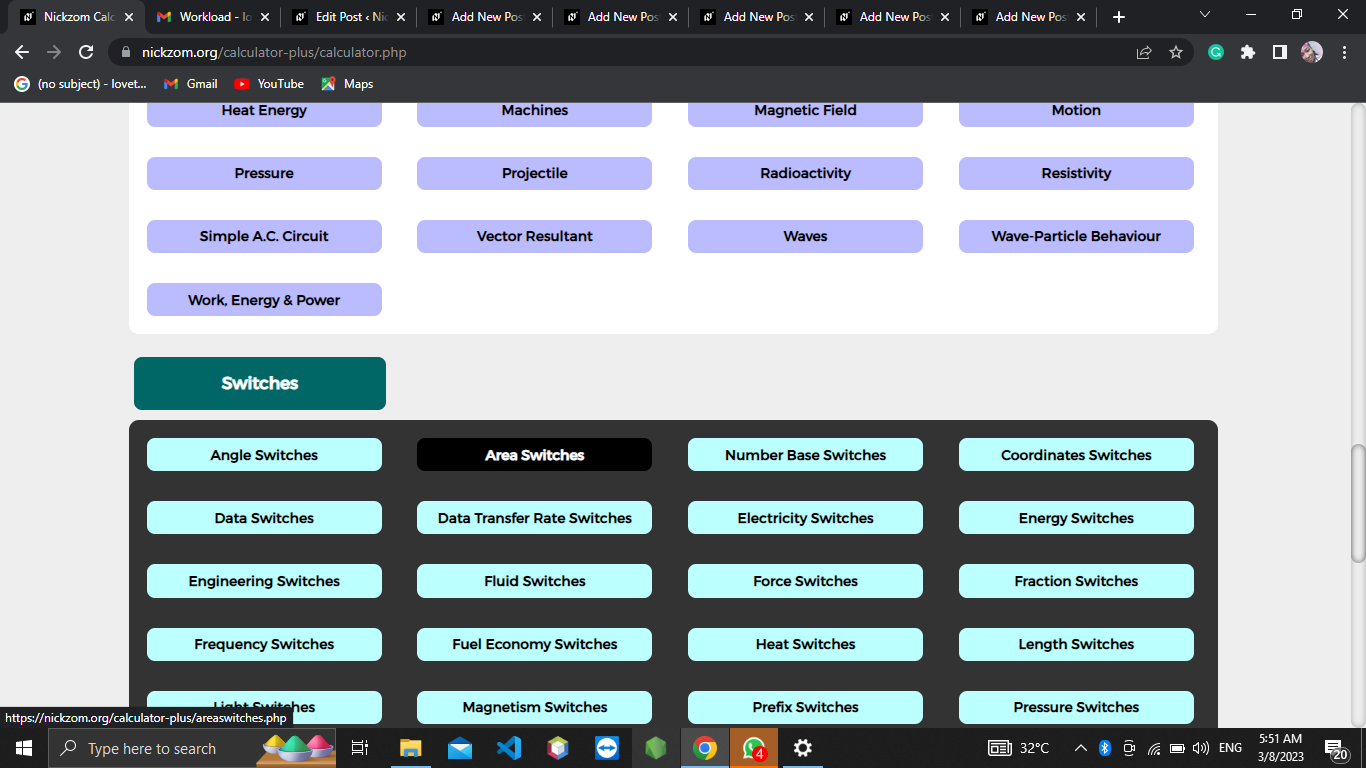The screenshot below displays the page or activity to enter your value, to get the answer for the conversion of square yards to square inches according to the respective parameter which is the Unit Value.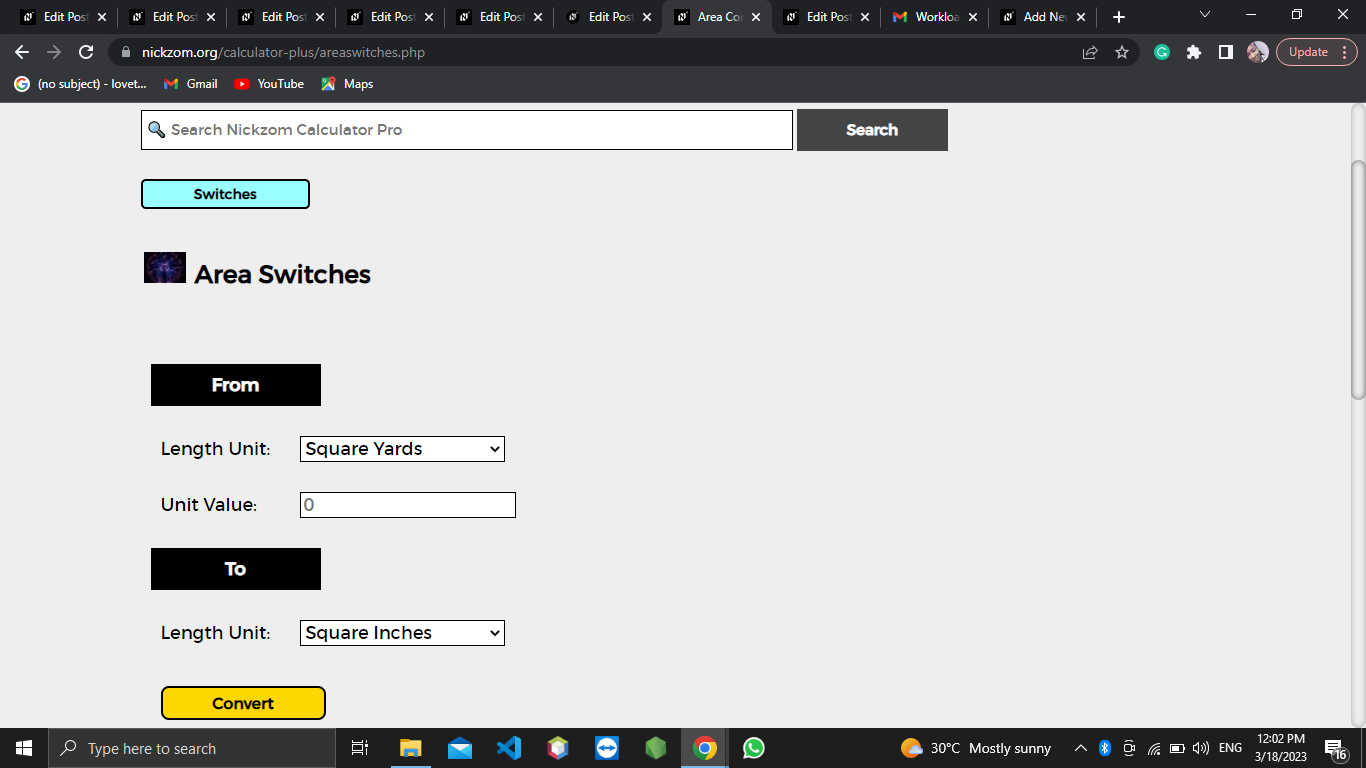Now, enter the value appropriately and accordingly for the parameter as required by the Unit Value is 3.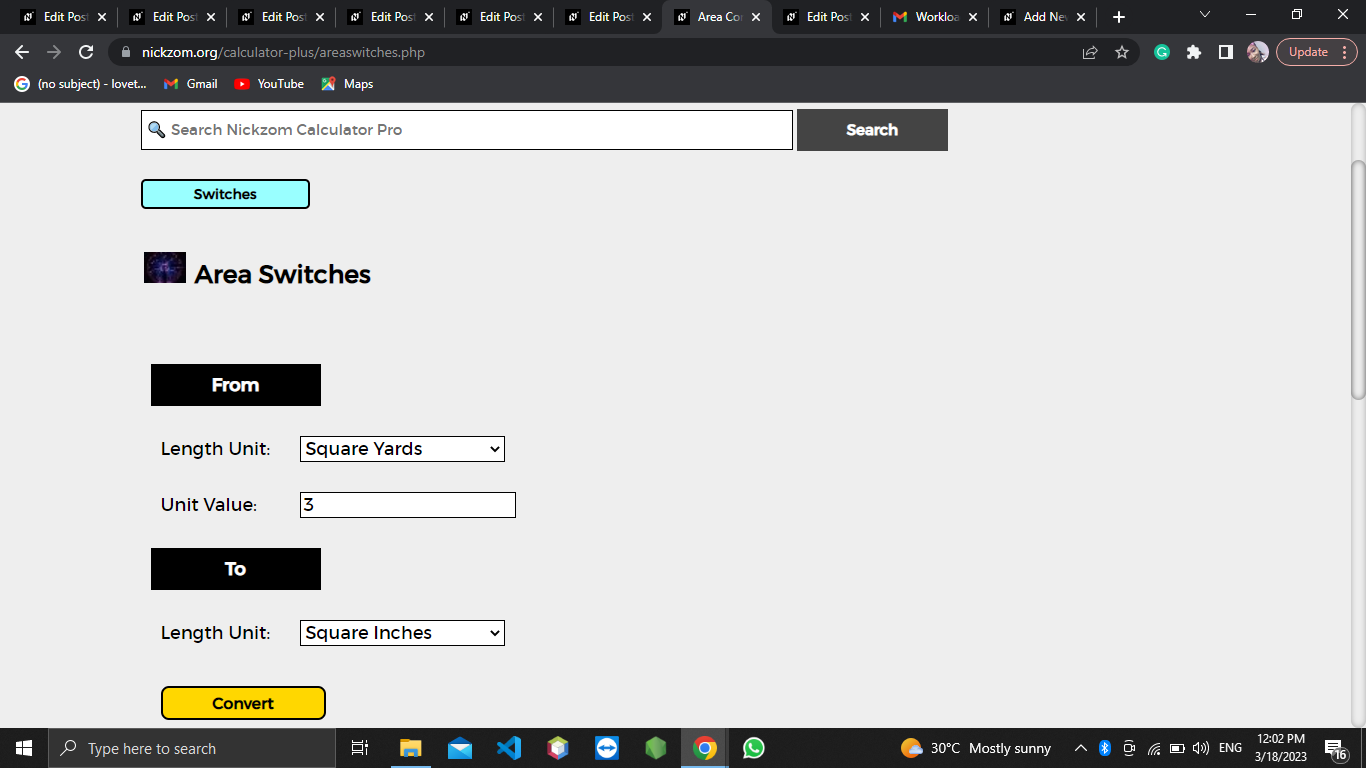Finally, Click on Calculate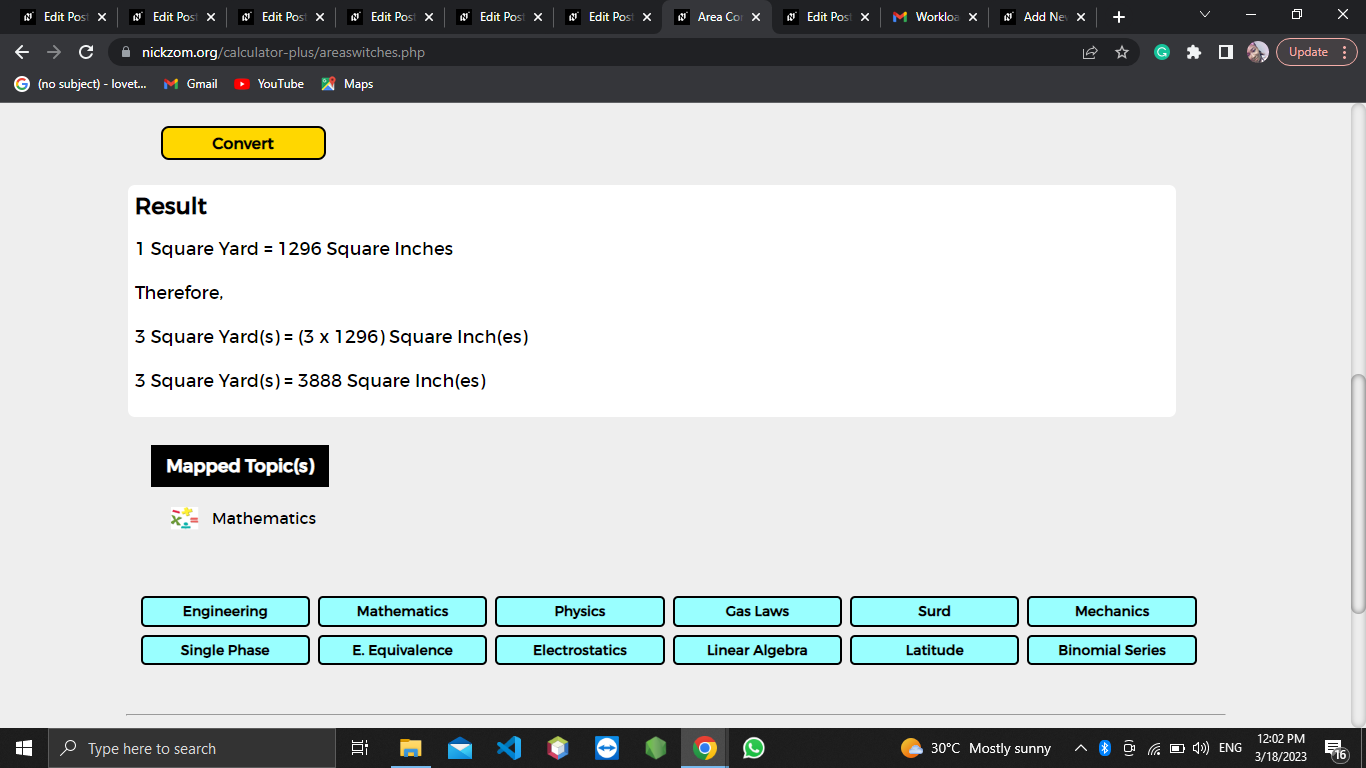As you can see from the screenshot above, Nickzom Calculator– The Calculator Encyclopedia solves the conversion of square yards to square inches and presents the formula, workings, and steps too.